# CBSE Class 10 Maths Exam 2021: Chapter-Wise Important Formulas, Theorems & Properties for Quick Revision

We have collated all important formulas from all chapters of CBSE Class 10 Maths. Important terms and properties which are useful in the Class 10 Maths calculations are also provided in this article for quick revision before the exam.CBSE 10th Maths Important formulas, theorems and properties

Mathematics is one such subject that often gives nightmares to students. While Maths is a little tricky, it is not difficult. It just requires a thorough understanding of the concepts, regular practice and a good hold on all important formulas to score high in the Maths subject.

To help students get all important formulas, theorems and properties in one place, we have collated the chapter-wise formulas along with important terms & properties occurring in Class 10 Maths.  Students must grasp all the formulas and theorems included in chapters like Triangles, Polynomials, Coordinate Geometry, Trigonometry and Mensuration as these chapters carry high weightage for Maths Board Exam 2021.

1. Real Numbers:

Euclid’s Division Algorithm (lemma): According to Euclid’s Division Lemma if we have two positive integers a and b, then there exist unique integers q and r  such that a = bq + r, where 0 ≤ r ≤ b. (Here, a = dividend, b = divisor, q = quotient and r = remainder.)

2. Polynomials:

(i) (a + b)2 = a2 + 2ab + b2

(ii) (a – b)2  = a2 – 2ab + b2

(iii) a2 – b= (a + b) (a – b)

(iv) (a + b)3  = a3 + b3 + 3ab(a + b)

(v) (a – b)3  =  a3 – b3 – 3ab(a – b)

(vi) a3 + b3 = (a + b) (a– ab + b2)

(vii) a3 – b3  = (a – b) (a+ ab + b2)

(viii) a4 – b4 = (a2)2 – (b2)2 = (a2 + b2) (a2 – b2) = (a2 + b2) (a + b) (a – b)

(ix) (a + b + c) 2  = a2 + b2 + c2 + 2ab + 2bc + 2ac

(x) (a + b – c) 2  =  a2 + b2 + c2 + 2ab – 2bc – 2ca

(xi) (a – b + c)2  = a2 + b2 + c2 – 2ab – 2bc + 2ca

(xii) (a – b – c)2  = a2 + b2 + c2 – 2ab +  2bc – 2ca

(xiii) a3 + b3 + c3 – 3abc  = (a + b + c)(a2 + b2 + c2 – ab – bc – ca)

3. Linear Equations in Two Variables:

For the pair of linear equations

a1 + b1y + c1 = 0  and  a2 + b2y + c2 = 0,

the nature of roots (zeroes) or solutions is determined as follows:

(i) If a1/a2 ≠ b1/b2 then we get a unique solution and the pair of linear equations in two variables are consistent. Here, the graph consists of two intersecting lines.

(i) If a1/a2 ≠ b1/b2 ≠ c1/c2, then there exists no solution and the pair of linear equations in two variables are said to be inconsistent. Here, the graph consists of parallel lines.

(iii) If a1/a2 = b1/b2 = c1/c2, then there exists infinitely many solutions and the pair of lines are coincident and therefore, dependent and consistent. Here, the graph consists of coincident lines.

4. Quadratic Equation:

For a quadratic equation, ax+ bx + c = 0• Sum of roots = –b/a
• Product of roots = c/a
• If roots of a quadratic equation are given, then the quadratic equation can be represented as:

x2 – (sum of the roots)x + product of the roots = 0

• If Discriminant > 0, then the roots the quadratic equation are real and unequal/unique.
• If Discriminant = 0, then the roots the quadratic equation are real and equal.
• If Discriminant < 0, then the roots the quadratic equation are imaginary (not real).
• Important Formulas – Boats and Streams
 (i) Downstream In water, the direction along the stream is called downstream. (ii) Upstream In water, the direction against the stream is called upstream. (iii) Let the speed of a boat in still water be u km/hr and the speed of the stream be v km/hr, then Speed downstream = (u + v) km/hr Speed upstream = (u – v) km/hr.

5. Arithmetic Progression:

• nth Term of an Arithmetic Progression: For a given AP, where a is the first term, d is the common difference, n is the number of terms, its nth term (an) is given as

a= a + (n−1)×d

• Sum of First n Terms of an Arithmetic Progression, Sn is given as: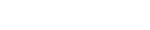6. Similarity of Triangles:

• If two triangles are similar then ratio of their sides are equal.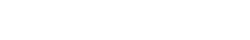• Theorem on the area of similar triangles: If two triangles are similar, then the ratio of the area of both triangles is proportional to the square of the ratio of their corresponding sides.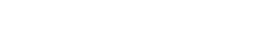7. Coordinate Gemetry:

• Distance Formulae: Consider a line having two point A(x1, y1) and B(x2, y2), then the distance of these points is given as: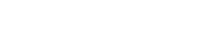• Section Formula: If a point p divides a line AB with coordinates A(x1, y1) and B(x2, y2), in ratio m:n, then the coordinates of the point p are given as: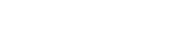• Mid Point Formula: The coordinates of the mid-point of a line AB with coordinates A(x1, y1) and B(x2, y2), are given as: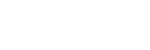• Area of a Triangle: Consider the triangle formed by the points A(x1, y1) and B(x2, y2) and C(x3, y3) then the area of a triangle is given as-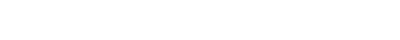8. Trigonometry:

In a right-angled triangle, the Pythagoras theorem states

(perpendicular )+ ( base )2 = ( hypotenuse )2

Important trigonometric properties: (with P = perpendicular, B = base and H = hypotenuse)

• SinA = P / H
• CosA = B / H
• TanA = P / B
• CotA = B / P
• CosecA = H / P
• SecA = H/B

Trigonometric Identities:

• sin2A + cos2A=1
• tan2A +1 = sec2A
• cot2A + 1= cosec2A

Relations between trigonometric identities are given below:Trigonometric Ratios of Complementary Angles are given as follows:

• sin (90° – A) = cos A
• cos (90° – A) = sin A
• tan (90° – A) = cot A
• cot (90° – A) = tan A
• sec (90° – A) = cosec A
• cosec (90° – A) = sec A

Values of Trigonometric Ratios of 0° and 90° are tabulated below:9. Circles:

Important properties related to circles:

• Equal chords of a circle are equidistant from the centre.
• The perpendicular drawn from the centre of a circle, bisects the chord of the circle.
• The angle subtended at the centre by an arc = Double the angle at any part of the circumference of the circle.
• Angles subtended by the same arc in the same segment are equal.
• To a circle, if a tangent is drawn and a chord is drawn from the point of contact, then the angle made between the chord and the tangent is equal to the angle made in the alternate segment.• The sum of opposite angles of a cyclic quadrilateral is always 180o.

Important formulas related to circles:• Area of a Segment of a Circle: If AB is a chord which divides the circle into two parts, then the bigger part is known as major segment and smaller one is called minor segment.Here, Area of the segment APB = Area of the sector OAPB – Area of ∆ OAB

10. Mensuration:

Check below the important formulas for areas and volumes of solids:11. Statistics:

For Ungrouped Data:

Mean: The mean value of a variable is defined as the sum of all the values of the variable divided by the number of values.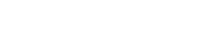Median: The median of a set of data values is the middle value of the data set when it has been arranged in ascending order.  That is, from the smallest value to the highest value.
Median is calculated as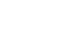Where n is the number of values in the data. If the number of values in the data set is even, then the median is the average of the two middle values.
Mode: Mode of a statistical data is the value of that variable which has the maximum frequency

For Grouped Data:

Mean: If x1, x2, x3,……xn are observations with respective frequencies f1, f2, f3,…..fn then mean is given as:Median: For the given data, we need to have class interval, frequency distribution and cumulative frequency distribution. Then, median is calculated as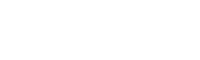Where
l = lower limit of median class,
n = number of observations,
cf = cumulative frequency of class preceding the median class,
f = frequency of median class,
h = class size (assuming class size to be equal)

Mode: Modal class: The class interval having highest frequency is called the modal class and Mode is obtained using the modal class.Where
l = lower limit of the modal class,
h = size of the class interval (assuming all class sizes to be equal),
f1 = frequency of the modal class,
f0 = frequency of the class preceding the modal class,
f2 = frequency of the class succeeding the modal class.

12. Probability: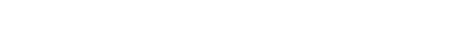Understanding the basic concepts and learning all the important formulas is extremely sufficient to pass the Maths exam with flying colours. If you know the formulas very well then it will not take much time for you to solve questions in the exam paper. So, keep practicing with the list of important formulas given above in this article.